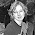## miércoles, 29 de mayo de 2013

### Assignment 6. Image Compression

For this week, we were assigned to implement a transform of compression image. In this oportunitty I apply the wavlets for compress the image and show the results.

Repositoty: https://gist.github.com/vane90/5670878

Pywavelets :
I used pywavelets its a free open source wavelet transform software in python, it is very easy to use if I worked with 2D Forward and Inverse Discrete Wavelet Transform  but it includes, many other features you can look for more information about this library in the page official.

Instalation
The first thing we need to do in order to work with this tool is to install the Pack needed to be imported to our python script.

The pywt is an module needed to be able to work with the program that will show. It can be installed by using the next line code on any linux terminal

Why does the compression of images?
To compress images, we can reduce the redundancy, or the frequency of the image data in order to be able to store or transmit data in an efficient way.

Wavelet analysis can often compress or delete noise without appreciable degradation.

For this it can considere two methods of data compression and are classified into:
• Lossless: it is the compression without loss of information
• Lossy: it is the compression with loss of information.
Transformed Wavelet:
This transform is efficient for local analysis of non-stationary signals quick transitoriness and, like the Fourier transfor with window, maps the signal into a representation. The temporary aspect of the signal is preserved.

The Wavelets, bases of the transformed Wavelet functions, are generated from a basic Wavelet function, through translations and dilations. These functions allow to reconstruct the original signal through the transformed Wavelet inverse in any case.

Wavelet analysis is to decompose a signal into mobile scaled versions of the original wavelet ("mother"). The comparison allows to obtain coefficients that are susceptible of interpretation and subsequent manipulation.

Descomposition wavelet:
In this case I use the transform of discrete wavelets (DWT): it will be used this transformed for a new representation of the image, more appropriate for the compression process

We choose a wavelet to work with it and we apply it, this will generate a array of coefficients, known as wavelet coefficients which are four:

• csA: Approximation Coefficient
• csH:horizontal Coefficient
• csV: vertical Coefficient
• csD: diagonal Coefficient

We can then apply each of the coefficients to create new images that will show each detail contained in each coefficient.

This are the process for the compression to the image:

Step 1: This procces Convert the imagen to grey scale
First apply gray scale that already has been handled previously, this consists of adding the value of r, g and b of each pixel and take your average, finally is assigned the same value to each r, g, b).

Step 2. Subsequently generated an array of the image to grayscale (in the new image became smaller, here the array is created for full-size image width by height):

Step3. Then we call the function coefficients, where we apply the function of the DWT (Discrete Wavelet transformed).

Use the dwt2 () function to apply this transform for 2 dimensions, it requires 2 parameters to work:
pywt.dwt2 (data, wavelet)

Data: Array with the information of the pixels.

Wavelet: We choose a wavelet in this case "haar".

Step4. Create an image for each coefficient. A first test print the array that returned coefficients are made and saw that numbers returning negative and greater than 255.

I need to apply a filter because the generating arrays from the coefficients contain negative numbers and numbers that pass 255 so this cant be included on and image, what we do is to create a filter any negative value will be 0 and any value that pass 255 will be 255 any other value will remain the same, after fixing the array we apply it to a new image generating the coefficient images.

Step5. The images are created

Approximation Coefficient

vertical Coefficient

Diagonal Coefficient

6.Compressed Image

Compression level

Differences of what was the capacity of the compressed image to the original (image in grayscale) reduction:

Test 2:

Approximation Coefficient

Horizontal
Vertical
Diagonal
Compress

Reference:

Filip Wasilewski, "PyWavelets - Discrete Wavelet Transform in Python", 31 de Agosto de 2012. [Accessed:  27 de Mayo de 2013], in http://www.pybytes.com/pywavelets/

TEORÍA DE WAVELETS, [Accessed: May 28th, 2013]
http://catarina.udlap.mx/u_dl_a/tales/documentos/lem/hernandez_d_m/capitulo2.pdf

Wavelet, [Accessed: May 27th,2013]
http://elisa.dyndns-web.com/~elisa/teaching/comp/vision/wavelet.pdf

#### 1 comentario:

1.Para cada ejemplo sería bueno ver el tiempo de ejecución y la tasa de compresión alcanzada. No vale copiar texto de otros lados al reporte. El formato de las referencias ocupa mejora. Van 5 pts por el reporte.

El código no es muy original (o sea, no hay una aportación propia en el método). Ocuparía también más comentarios. Van 6 pts por ello.